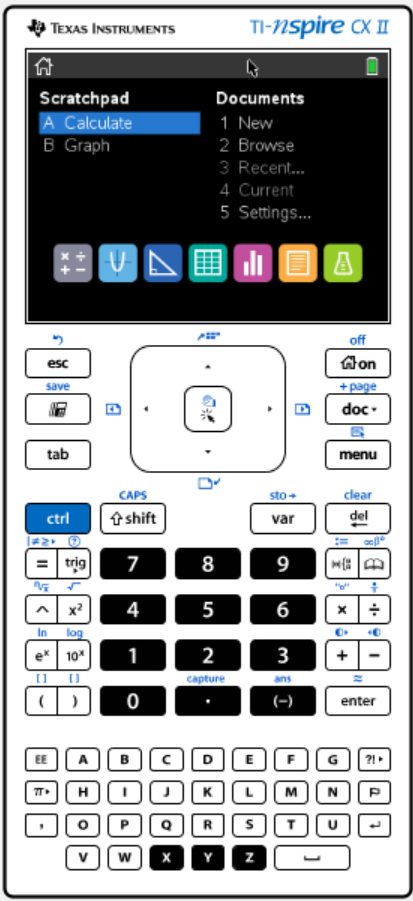Preview
Length: 10 minutes

## Introduction to your TI Npsire Calculator

This video will explain the introdcution to this course!

It is a huge advantage to have this calculator but it is no good if you are not confident in using it. There are alot of features on this calculator that will allow you to do some Math that you have always been doing but now the calculator will do it for you.

This course will help you to prepare for a Mathematics exam which allows the use of a TI – Nspire calculator.

This course is aimed at students who want to learn the basics of their TI Nspire calculator. While you will touch on some mathematical concepts it is assumed that you have an average level of mathematics and have studied mathematics to a GCSE or equivalent level.This is the screen of your TI Nspire calculator. It is divided up into sections with each section to help you with different topics. It can be a little bit confusing at the beginning to figure out which functions are for which sections. I will break this down as we progress through this course.

Back to: TI – NSPIRE Calculator Course > Introduction